×
Get Full Access to Contemporary Abstract Algebra - 8 Edition - Chapter 12 - Problem 36e
Get Full Access to Contemporary Abstract Algebra - 8 Edition - Chapter 12 - Problem 36e

×

# Let m and n be positive integers and let k be the leastISBN: 9781133599708 52

## Solution for problem 36E Chapter 12

Contemporary Abstract Algebra | 8th Edition

• Textbook Solutions
• 2901 Step-by-step solutions solved by professors and subject experts
• Get 24/7 help from StudySoup virtual teaching assistantsContemporary Abstract Algebra | 8th Edition

4 5 1 372 Reviews
30
0
Problem 36E

Problem 36E

Let m and n be positive integers and let k be the least common multiple of m and n. Show that mZ nZ = kZ.

Step-by-Step Solution:

36. Let m and n be positive integers and let k be the least common multiple of m and n. Show that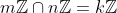.

Step By Step Solution

Step 1 of 2

Given k is the least common multiple of m and n.

Also we know any set of the form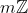is ideal, soandare ideals.

(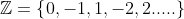the set of integers. If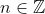is any integer, we writefor the set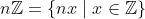.)

Step 2 of 2

##### ISBN: 9781133599708

Unlock Textbook Solution